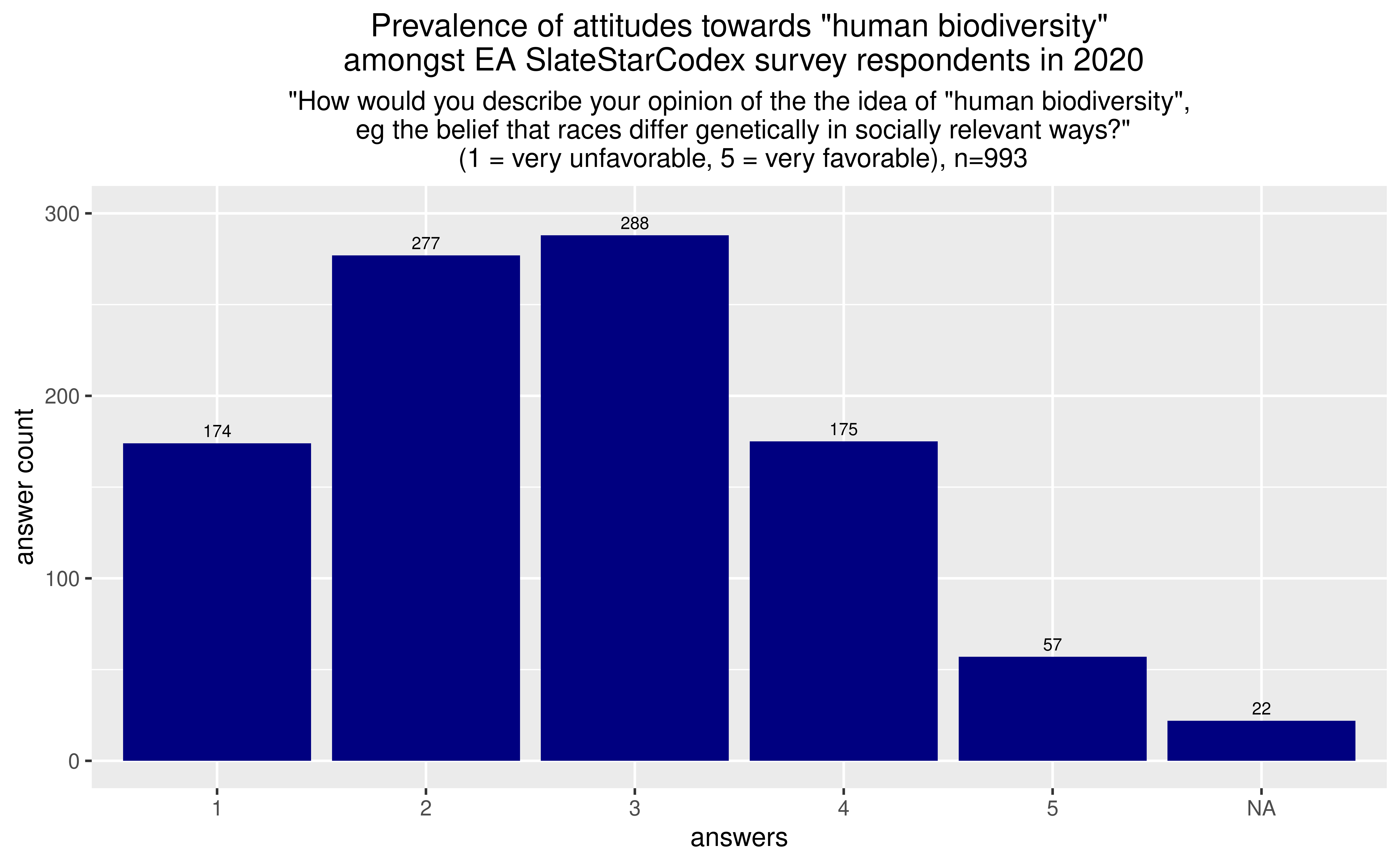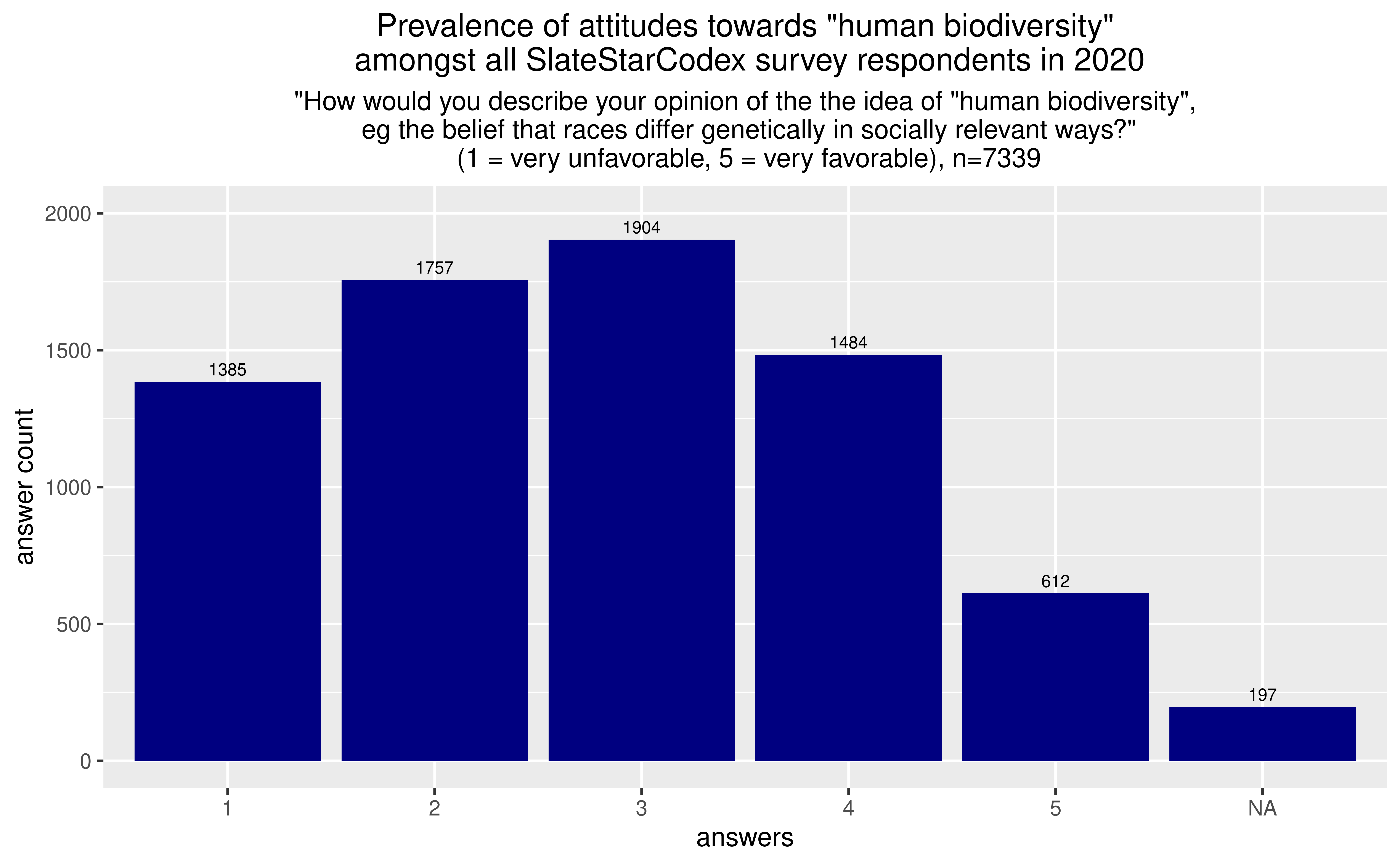# Prevalence of belief in “human biodiversity” amongst self-reported EA respondents in the 2020 SlateStarCodex Survey

Note: This post presents some data which might inform downstream questions, rather than providing a fully cooked perspective on its own. For this reason, I have tried to not really express many opinions here. Readers might instead be interested in more fleshed out perspectives on the Bostrom affair, e.g., here in favor or here against.

## Graph## Discussion

### Selection effects

I am not sure whether EAs who answered the EA forum are a representative sample of all EAs. It might not be, if SSC readers have shared biases and assumptions distinct from those of the EA population as a whole. That said, raw numerical numbers will be accurate, e.g., we can say that “at least 57 people who identified as EAs in 2020 strongly agree with the human biodiversity hypothesis”.

### Question framing effects

I think the question as phrased is likely to overestimate belief in human biodiversity, because the phrasing seems somewhat inocuous, and in particular because “biodiversity” has positive mood affiliation. I think that fewer people would answer positively to a less inocuous sounding version, e.g., “How would you describe your opinion of the the idea of "human biodiversity”,\n eg the belief that some races are genetically stupider than others? (1 = very unfavorable, 5 = very favorable)“.

For a review of survey effects, see A review of two books on survey-making.

### Interpreting as a probability

This isn’t really all that meaningful, but we can assign percentages to each answer as follows:

• 1: 5%
• 2: 20%
• 3: 50%
• 4: 80%
• 5: 95%
• NA: 50%

The above requires a judgment call to assign probabilities to numbers in a Likert scale. In particular, I am making the judgment call that 1 and 5 correspond to 5% and 95%, rather than e.g., 0% and 100%, or 1% and 99%, based on my forecasting experience.

And then we can calculate an implicit probability as follows

``````( 174 * 0.03 + 227 * 0.2 + 288 * 0.5 + 175 * 0.8 + 57 * 0.95 + 22 * 0.5) / 993
``````

The above calculation outputs 0.4025…, which, in a sense, means that SSC survey respondents which self-identified as EA assigned, as a whole, a 40% credence to the human biodiversity hypothesis.

### Comparison with all SSC respondents## Code to replicate this

In an R runtime, run:

``````## Libraries
library(ggplot2)

setwd("/home/loki/Documents/core/ea/fresh/misc/ea-hbd") ## change to the folder in your computer

## Restrict analysis to EAs
data_EAs <- data[data["EAID"] == "Yes",]
View(data_EAs)
n=dim(data_EAs)
n

## Find biodiversity question
colnames(data_EAs)
colnames(data_EAs)

## Process biodiversity question for EAs
tally <- list()
tally\$options = c(1:5, "NA")
tally\$count = sapply(tally\$options, function(x){ sum(data_EAs == x, na.rm = TRUE) })
tally\$count = sum(is.na(data_EAs))
tally\$count

tally = as.data.frame(tally)
tally

## Plot prevalence of belief within EA

titulo='Prevalence of attitudes towards "human biodiversity"\n amongst EA SlateStarCodex survey respondents in 2020'
subtitulo='"How would you describe your opinion of the the idea of "human biodiversity",\n eg the belief that races differ genetically in socially relevant ways?"\n (1 = very unfavorable, 5 = very favorable), n=993'

(ggplot(data = tally, aes(x =options, y = count)) +
geom_histogram(
stat="identity",
position= position_stack(reverse = TRUE),
fill="navyblue"
))+
scale_y_continuous(limits = c(0, 300))+
labs(
title=titulo,
subtitle=subtitulo,
legend.title = element_blank(),
legend.text.align = 0
)+
theme(
legend.title = element_blank(),
plot.subtitle = element_text(hjust = 0.5),
plot.title = element_text(hjust = 0.5),
legend.position="bottom"
) +
geom_text(aes(label=count,  size = 2), colour="#000000",size=2.5, vjust = -0.5)

height=5
width=height*(1+sqrt(5))/2

ggsave("q_hbd_EAs.png" , units="in", width=width, height=height, dpi=800)

## Process biodiversity question for all SSC respondents
tally_all_ssc <- list()
tally_all_ssc\$options = c(1:5, "NA")
tally_all_ssc\$count = sapply(tally_all_ssc\$options, function(x){ sum(data == x, na.rm = TRUE) })
tally_all_ssc\$count = sum(is.na(data))
tally_all_ssc\$count

tally_all_ssc = as.data.frame(tally_all_ssc)
tally_all_ssc
tally
## Plot

titulo='Prevalence of attitudes towards "human biodiversity"\n amongst all SlateStarCodex survey respondents in 2020'
subtitulo='"How would you describe your opinion of the the idea of "human biodiversity",\n eg the belief that races differ genetically in socially relevant ways?"\n (1 = very unfavorable, 5 = very favorable), n=993'

(ggplot(data = tally_all_ssc, aes(x =options, y = count)) +
geom_histogram(
stat="identity",
position= position_stack(reverse = TRUE),
fill="navyblue"
))+
scale_y_continuous(limits = c(0, 2000))+
labs(
title=titulo,
subtitle=subtitulo,
legend.title = element_blank(),
legend.text.align = 0
)+
theme(
legend.title = element_blank(),
plot.subtitle = element_text(hjust = 0.5),
plot.title = element_text(hjust = 0.5),
legend.position="bottom"
) +
geom_text(aes(label=count,  size = 2), colour="#000000",size=2.5, vjust = -0.5)

height=5
width=height*(1+sqrt(5))/2

ggsave("q_hbd_all.png" , units="in", width=width, height=height, dpi=800)
``````

The file 2020ssc_public.csv is no longer available in the SSC blogpost, but it can easily be created from the .xlsx file, or I can make it available for a small donation to the AMF.